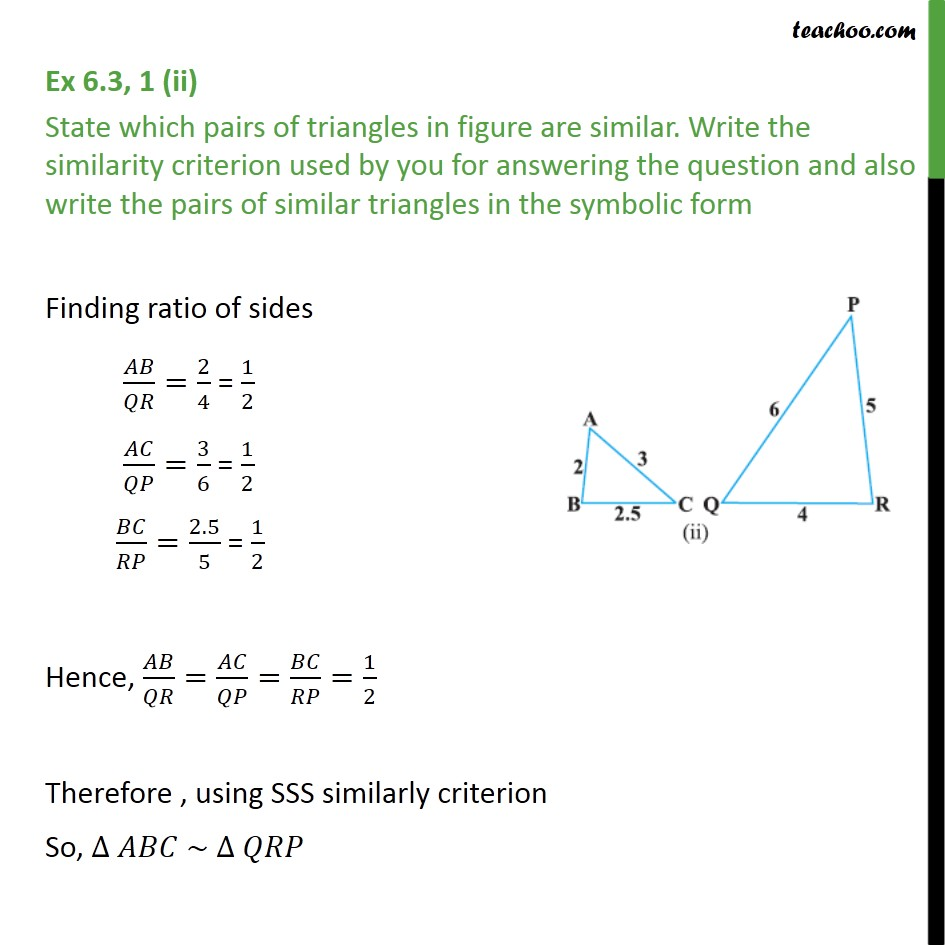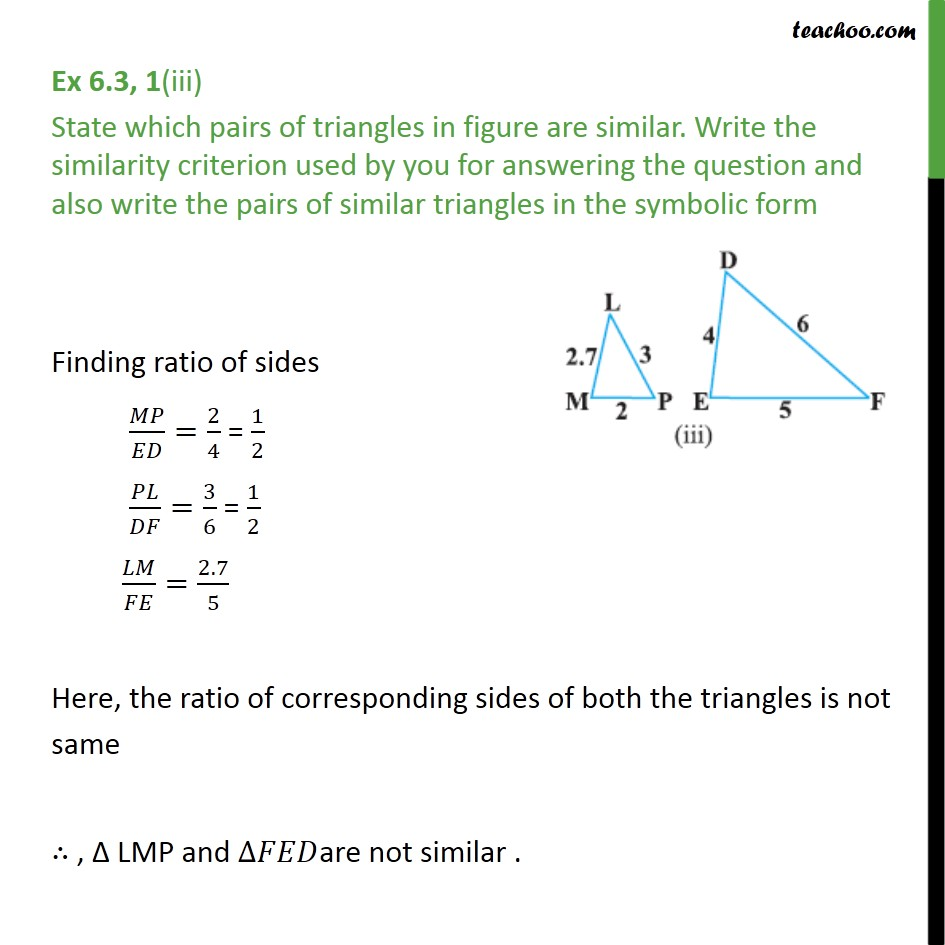1. Chapter 6 Class 10 Triangles (Term 1)
2. Concept wise
3. SSS Similarity

Transcript

Ex 6.3, 1 (ii) State which pairs of triangles in figure are similar. Write the similarity criterion used by you for answering the question and also write the pairs of similar triangles in the symbolic form Finding ratio of sides 𝐴𝐵/𝑄𝑅=2/4 = 1/2 𝐴𝐶/𝑄𝑃=3/6 = 1/2 𝐵𝐶/𝑅𝑃=2.5/5 = 1/2 Hence, 𝐴𝐵/𝑄𝑅=𝐴𝐶/𝑄𝑃=𝐵𝐶/𝑅𝑃=1/2 Therefore , using SSS similarly criterion So, ∆ 𝐴𝐵𝐶 ∼ ∆ 𝑄𝑅𝑃 Ex 6.3, 1(iii) State which pairs of triangles in figure are similar. Write the similarity criterion used by you for answering the question and also write the pairs of similar triangles in the symbolic form Finding ratio of sides 𝑀𝑃/𝐸𝐷=2/4 = 1/2 𝑃𝐿/𝐷𝐹=3/6 = 1/2 𝐿𝑀/𝐹𝐸=2.7/5 Here, the ratio of corresponding sides of both the triangles is not same ∴ , Δ LMP and ∆𝐹𝐸𝐷are not similar .

SSS Similarity